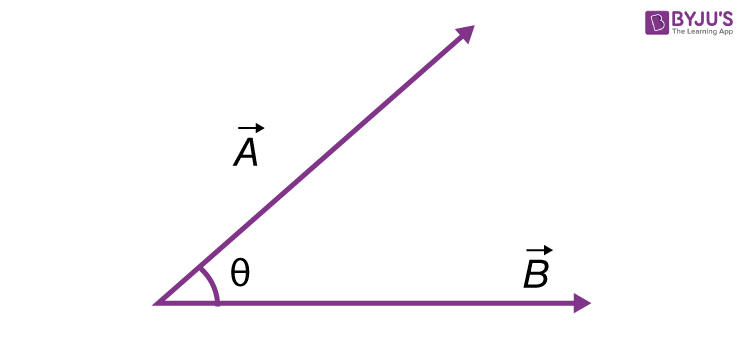Checkout JEE MAINS 2022 Question Paper Analysis : Checkout JEE MAINS 2022 Question Paper Analysis :

# Scalar And Vector Products

Scalar products and vector products are two ways of multiplying two different vectors which see the most application in physics and astronomy. The scalar product of two vectors is defined as the product of the magnitudes of the two vectors and the cosine of the angles between them.

## Scalar Product

“Scalar products can be found by taking the component of one vector in the direction of the other vector and multiplying it with the magnitude of the other vector”. It can be defined as:

Scalar product or dot product is an algebraic operation that takes two equal-length sequences of numbers and returns a single number.

This can be represented as follows:$$\begin{array}{l}Scalar\,product\,of \, \vec{A}.\vec{B}=ABcos\Theta\end{array}$$
• $$\begin{array}{l}\vec{A}\,denotes\,the\,vector\end{array}$$
• $$\begin{array}{l}A\,denotes\,the\,magnitude\,of\,vector\, \vec{A}\end{array}$$

The scalar product is also termed as the dot product or inner product and remember that scalar multiplication is always denoted by a dot.

If the same vectors are expressed in the form of unit vectors i, j and k along the axis x, y and z respectively, the scalar product can be expressed as follows:

$$\begin{array}{l}\vec{A}.\vec{B}=A_{X}B_{X}+A_{Y}B_{Y}+A_{Z}B_{Z}\end{array}$$

Where,

• $$\begin{array}{l}\vec{A}=A_{X}\hat{i}+A_{Y}\hat{j}+A_{Z}\hat{k}\end{array}$$
• $$\begin{array}{l}\vec{B}=B_{X}\hat{i}+B_{Y}\hat{j}+B_{Z}\hat{k}\end{array}$$

### Matrix Representation of Scalar Products

It is useful to represent vectors as row or column matrix, instead of as the above unit vectors. If we treat vectors as column matrices of their x, y and z components, then the transposes of these vectors would be row matrices.

$$\begin{array}{l}Therefore\,the\,vectors\, \vec{A} \, and \, \vec{B} \, would\, look\, like:\end{array}$$
$$\begin{array}{l}[\vec{A^T}=A_XA_YA_Z]\end{array}$$
$$\begin{array}{l}\vec{B}=\begin{bmatrix} B_X\\ B_Y\\ B_Z \end{bmatrix}\end{array}$$
The matrix product of these 2 matrices will give us the scalar product of the 2 matrices which is the sum of corresponding spatial components of the given 2 vectors, the resulting number will be the scalar product of vector A and vector B.

$$\begin{array}{l}\begin{bmatrix} A_X &A_Y &A_Z \end{bmatrix}\begin{bmatrix} B_X\\ B_Y\\ B_Z \end{bmatrix}=A_XB_X+A_YB_Y+A_ZB_Z=\vec{A}.\vec{B}\end{array}$$

## Vector Product

The magnitude vector product of two given vectors can be found by taking the product of the magnitudes of the vectors times the sine of the angle between them. It can be defined as:

Vector product or cross product is a binary operation on two vectors in three-dimensional space.

The magnitude of the vector product can be represented as follows:

$$\begin{array}{l}\vec{A}×\vec{B}=A\;BSin\theta\end{array}$$

Remember the above equation is only for the magnitude, for the direction of the vector product, the following expression is used,

$$\begin{array}{l}\vec{A}×\vec{B}=\vec{i}(A_YB_Z-A_ZB_Y)-\vec{j}(A_XB_Z-A_ZB_X)+\vec{k}(A_XB_Y-A_YB_X)\end{array}$$

[The above equation gives us the direction of the vector product]

### Vector Products Represented by Determinants

$$\begin{array}{l}\vec{A}×\vec{B}=\begin{vmatrix} \vec{i} &\vec{j} &\vec{k} \\ \vec{A_X}&\vec{A_Y} &\vec{A_Z} \\ \vec{B_X}&\vec{B_Y} &\vec{B_Z} \end{vmatrix}\end{array}$$

Now the above determinant can be solved as follows:

$$\begin{array}{l}\vec{A}×\vec{B}=\vec{i}(A_YB_Z-A_ZB_Y)-\vec{j}(A_XB_Z-A_ZB_X)+\vec{k}(A_XB_Y-A_YB_X)\end{array}$$

Application of scalar and vector products are countless especially in situations where there are two forces acting on a body in a different direction.

Example:

Calculation of the magnetic force acting on a moving charge in a magnetic field, other applications include determining the net force on a body.

## Frequently Asked Questions – FAQs

### Define the scalar product of two vectors.

The scalar product of two vectors is defined as the product of the magnitudes of the two vectors and the cosine of the angles between them.

### What is the scalar product also known as?

The scalar product is also known as the dot product or inner product.

### Define vector product.

A vector product or cross product is a binary operation on two vectors in three-dimensional space.

### How is the magnitude of the vector product represented?

The magnitude of the vector product is represented as
$$\begin{array}{l}\vec{A}×\vec{B}=A\;BSin\theta\end{array}$$

### How is the scalar product represented?

The scalar product is represented as:
$$\begin{array}{l}\vec{A}.\vec{B}=ABcos\theta\end{array}$$
.
Test your knowledge on Scalar And Vector Products